# Rational numbers: Intro (Math 0)

In order to get the most out of this page, you probably want a good grasp of the integers first.

“Rational number” is a phrase mathematicians use for the idea of a “fraction”. Here, we’ll go through what a fraction is and why we should care about them.

# What is a fraction?

So far, we’ve met the integers: whole numbers, which can be either bigger than $$0$$ or less than $$0$$ (or the very special $$0$$ itself). The natural numbers can count the number of cows I have in my possession; the integers can also count the number of cows I have after I’ve given away cows from having nothing, resulting in anti-cows.

Suppose I have two apples. noteI’m terrible at drawing, so my apples look suspiciously like circles.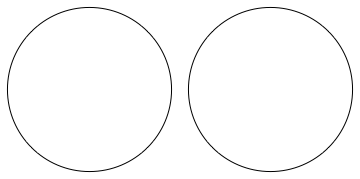What if I chopped one of the apples into two equally-sized pieces? (And now you know why we stopped talking about cows.)

Now what I have is a whole apple, and… another apple which is in two pieces.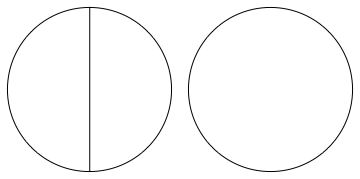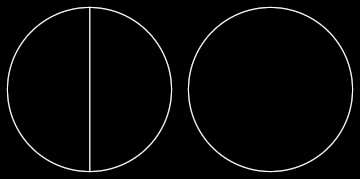Let’s imagine now that I chop one of the pieces itself into two pieces, and for good measure I chop my remaining whole apple into three pieces.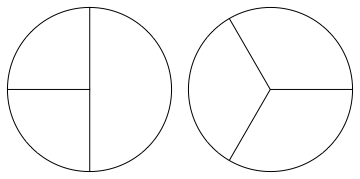I still have the same amount of apple as I started with—I haven’t eaten any of the pieces or anything—but now it’s all in funny-sized chunks.

Now I’ll eat one of the smallest chunks. How many apples do I have now?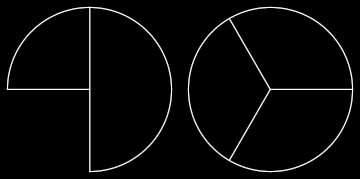I certainly don’t just have one apple, because three of the chunks I’ve got in front of me will together make an apple; and I’ve also got some chunks left over once I’ve done that. But I can’t have two apples either, because I started with two and then I ate a bit. Mathematicians like to be able to compare things, and if I forced you to make a comparison, you could say that I have “more than one apple” but “fewer than two apples”.

If you’re happy with that, then it’s a reasonable thing to ask: “exactly how much apple do I have?”. And the mathematician will give an answer of “one apple and three quarters”. “One and three quarters” is an example of a rational number or fraction: it expresses a quantity that came from dividing some number of things into some number of equal parts, then possibly removing some of the parts. noteI’ve left out the point that just as you moved from the counting numbers to the integers, thereby allowing you to owe someone some apples, so we can also have a negative rational number of apples. We’ll get to that in time.

# The basic building block

From a certain point of view, the building block of the natural numbers is just the number $$1$$: all natural numbers can be made by just adding together the number $$1$$ some number of times. (If I have a heap of apples, I can build it up just from single apples.) The building block of the integers is also the number $$1$$, because if you gave me some apples notewhich perhaps I’ve now eaten so that I owe you some apples, you might as well have given them to me one by one.

Now the rationals have building blocks too, but this time there are lots and lots of them, because if you give me any kind of “building block”—some quantity of apple—I can always just chop it into two pieces and make a smaller “building block”. (This wasn’t true when we were confined just to whole apples, as in the natural numbers! If I can’t divide up an apple, then I can’t make any quantity of apples smaller than one apple. noteExcept no apples at all.)

It turns out that a good choice of building blocks is “one piece, when we divide an apple into several equally-sized pieces”. If we took our apple, and divided it into five equal pieces, then the corresponding building-block is “one fifth of an apple”: five of these building blocks makes one apple. To a mathematician, we have just made the rational number which is written $$\frac{1}{5}$$.

Similarly, if we divided our apple instead into six equal pieces, and take just one of the pieces, then we have made the rational number which is written $$\frac{1}{6}$$.

The (positive) rational numbers are just whatever we could make by taking lots of copies of building blocks.

# Examples

• $$1$$ is a rational number. It can be made with the building block that is just $$1$$ itself, which is what we get if we take an apple and divide it into just one piece—that is, making no cuts at all. Or, if you’re a bit squeamish about not making any cuts, $$1$$ can be made out of two halves: two copies of the building block that results when we take an apple and cut it into two equal pieces, taking just one of the pieces. (We write $$\frac{1}{2}$$ for that half-sized building block.)

• $$2$$ is a rational number: it can be made out of two lots of the $$1$$-building-block, or indeed out of four lots of the $$\frac{1}{2}$$-building-block.

• $$\frac{1}{2}$$ is a rational number: it is just the half-sized building block itself.

• If we took the apple and instead cut it into three pieces, we obtain a building block which we write as $$\frac{1}{3}$$; so $$\frac{1}{3}$$ is a rational number.

• Two copies of the $$\frac{1}{3}$$-building-block makes the rational number which we write $$\frac{2}{3}$$.

• Five copies of the $$\frac{1}{3}$$-building-block makes somewhat more than one apple. Indeed, three of the building blocks can be put together to make one full apple, and then we’ve got two building blocks left over. We write the rational number represented by five $$\frac{1}{3}$$-building-blocks as $$\frac{5}{3}$$.

# Notation

Now you’ve seen the notation $$\frac{\cdot}{\cdot}$$ used a few times, where there are numbers in the places of the dots. You might be able to guess how this notation works in general now: if we take the blocks resulting when we divide an apple into “dividey-number”-many pieces, and then take “lots” of those pieces, then we obtain a rational number which we write as $$\frac{\text{lots}}{\text{dividey-number}}$$. Mathematicians use the words “numerator” and “denominator” for what I called “lots” and “dividey-number”; so it would be $$\frac{\text{numerator}}{\text{denominator}}$$ to a mathematician.

# Exercises

Can you give some examples of how we can make the number $$3$$ from smaller building blocks? (There are lots and lots of ways you could correctly answer this question.)

You already know about one way from when we talked about the natural numbers: just take three copies of the $$1$$-block. (That is, three apples is three single apples put together.)

Another way would be to take six half-sized blocks: $$\frac{6}{2}$$ is another way to write $$3$$.

Yet another way is to take fifteen fifth-sized blocks: $$\frac{15}{5}$$ is another way to write $$3$$.

If you want to mix things up, you could take four half-sized blocks and three third-sized blocks: $$\frac{4}{2}$$ and $$\frac{3}{3}$$ together make $$3$$.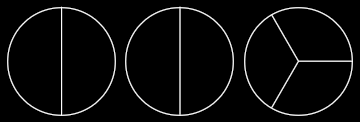<div><div>

If you felt deeply uneasy about the last of my possible solutions above, there is a good and perfectly valid reason why you might have done; we will get to that eventually. If that was you, just forget I mentioned that last one for now. If you were comfortable with it, that’s also normal.

How about making the number $$\frac{1}{2}$$ from smaller blocks?

Of course, you could start by taking just one $$\frac{1}{2}$$ block.

For a more interesting answer, you could take three copies of the sixth-sized block: $$\frac{3}{6}$$ is the same as $$\frac{1}{2}$$.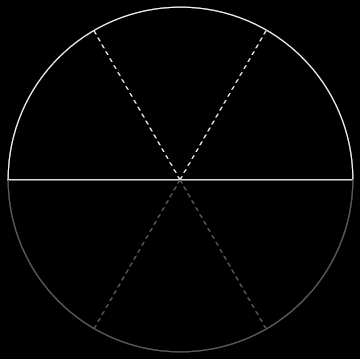Alternatively, five copies of the tenth-sized block: $$\frac{5}{10}$$ is the same as $$\frac{1}{2}$$.<div><div>

The way I’ve drawn the pictures might be suggestive: in some sense, when I’ve given different answers just now, they all look like “the same answer” but with different lines drawn on. That’s because the rational numbers (“fractions”, remember) correspond to answers to the question “how much?”. While there is always more than one way to build a given rational number out of the building blocks, the way that we build the number doesn’t affect the ultimate answer to the question “how much?”. $$\frac{5}{10}$$ and $$\frac{1}{2}$$ and $$\frac{3}{6}$$ are all simply different ways of writing the same underlying quantity: the number which represents the fundamental concept of “chop something into two equal pieces”. They each express different ways of making the same amount (for instance, out of five $$\frac{1}{10}$$-blocks, or one $$\frac{1}{2}$$-block), but the amount itself hasn’t changed.

# Going more general

Remember, from when we treated the integers using cows, that I can give you a cow (even if I haven’t got one) by creating a cow/​anti-cow pair and then giving you the cow, leaving me with an anti-cow. We count the number of anti-cows that I have by giving them a negative number.

We can do the same here with chunks of apple. If I wanted to give you half an apple, but I didn’t have any apples, I could create a half-apple/​half-anti-apple pair, and then give you the half-apple; this would leave me with a half-anti-apple.

We count anti-apples in the same way as we count anti-cows: they are negative.

See the page on subtraction for a much more comprehensive explanation; this page is more of a whistle-stop tour.

# Limitations

We’ve had the idea of building-blocks: as $$\frac{1}{n}$$, where $$n$$ was a natural number. Why should $$n$$ be just a natural number, though? We’ve already seen the integers; why can’t it be one of those? noteThat is, why not let it be negative?

As it turns out, we can let $$n$$ be an integer, but we don’t actually get anything new if we do. We’re going to pretend for the moment that $$n$$ has to be positive, because it gets a bit weird trying to divide things into three anti-chunks; this approach doesn’t restrict us in any way, but if you are of a certain frame of mind, it might just look like a strange and artificial boundary to draw.

However, you must note that $$n$$ cannot be $$0$$ (whatever your stance on dividing things into anti-chunks). While there is a way to finesse the idea of an anti-chunk noteAnd if you sit and think really hard for a long time, you might even come up with it yourself!, there is simply no way to make it possible to divide an apple into $$0$$ equal pieces. That is, $$\frac{1}{0}$$ is not a rational number (and you should be very wary of calling it anything that suggests it’s like a number—like “infinity”—and under no account may you do arithmetic on it).

# Summary

So far, you’ve met what a rational number is! We haven’t gone through how to do things with them yet, but hopefully you now understand vaguely what they’re there for: they express the idea of “dividing something up into parts”, or “sharing things out among people” (if I have two apples to split fairly among three people, I can be fair by chopping each apple into three $$\frac{1}{3}$$-sized building blocks, and then giving each person two of the blocks).

Next up, we will see how we can combine rational numbers together, eventually making a very convenient shorthand. noteThe study of this shorthand is known as “arithmetic”.

Parents: